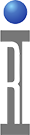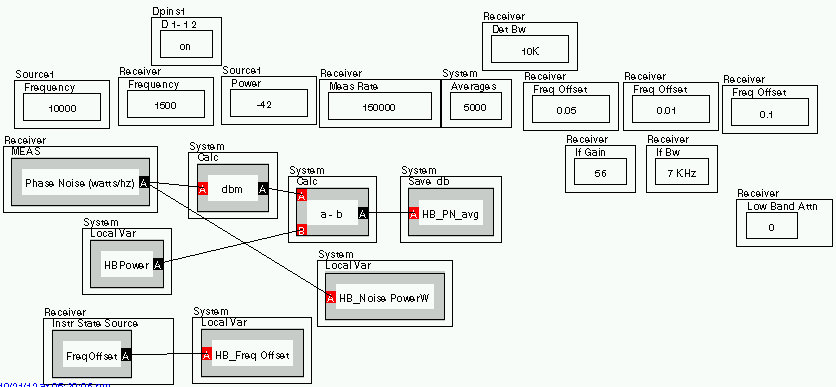Integrated phase noise can be calculated from spot phase noise measurements taken with an RI Receiver or the 8575A Phase noise TIM. Integrated phase noise is simply, the area under the curve calculated from linear vs linear results.

Here is a simple panel with an 8587 reciever that measures noise power in Watts/Hz at several offsets. The Noise power and the frequency offset are stored into LVs. The Main tone power is already in an LV. (HB Power)In a subsequent panel those values are brought into a standard 3 input calc button, And the result here is saved in degrees RMS.If using the 8575 Phase noise TIM. the Phase noise button will return a dBc/Hz, That will have to be expanded into two numbers that give the same ratio of power, to use this calculation. For instance 90 dBc/Hz = 10 log (P1/P2). For a nominal P1 of "1" calculate the P2, then use the two numbers P1 and P2 for the calc.

https://roos.com/docs/MFEY-8ZMQPA
ROOS INSTRUMENTS CONFIDENTIAL AND PROPRIETARY